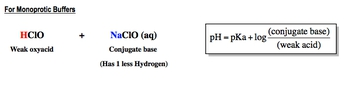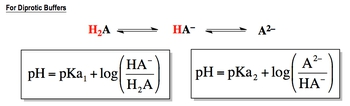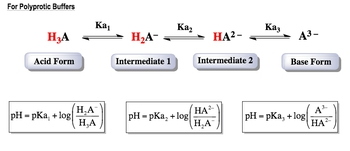## General Chemistry

Learn the toughest concepts covered in Chemistry with step-by-step video tutorials and practice problems by world-class tutors

18. Aqueous Equilibrium

# Titrations of Diprotic and Polyprotic Acids

A diprotic or polyprotic buffer can be approached in a way similar to monoprotic buffers. The key difference is that multiple pKa values will be involved.

Diprotic & Polyprotic Buffers
1
concept

## Diprotic & Polyprotic Buffers2m
Play a video:
So in this video, guys, we're gonna continue with our discussion of buffers. But now, looking at die product as well as Polly product buffers, Now we're gonna say here, Die product or Polly Product Buffer can be approached in a way similar to mono product buffers. The main difference, though, is with mono product acids. We're dealing with just one K value, but with di product buffers, we're dealing with two K values because there's too acidic hydrogen and with try product buffers, we're dealing with three K values because there's three acidic hydrogen now here, it says For mono product buffers, remember, buffer simply a weak acid. In this case we have Hi Paul Cloris Acid, which is a week oxy acid. And then we have sodium hypochlorite, which is its conjugate base. Remember, you're confident based normally has one less hydrogen and usually toe disguise the chart that's formed. They will replace that hydrogen we lost with a metal. When we have a buffer, we just use simply the Henderson household back equation. And remember what the Henderson Hasselbach equation. It's just pH equals P K, which remember, is negative log of K plus log of the conjugate base over the weak acid. Now, the units that go in here, they can be either mole arat e if we're given just polarity or they could be moles. We tend to use moles when they're giving us volume and molar ity. So, for example, if this that you had 50 miles off 500.100 Moeller hcl Oh, as the amount for your weak acid remember, polarity equals moles over leaders. So if they're giving you volume and polarity realize that if I multiply both sides by leaders here, we can see that moles equals leaders times more clarity. So you would divide the MLS here by 1000 to get leaders and then multiply them by their mole arat e to find your moles. Okay, so those are the units that we're gonna use their remember, if they're giving you just polarity, the units will be more clarity. But if they give you volume of a polarity there really asking you to find your moles, this is the basic set up. When it comes to mono product buffers. We'll continue onward with looking at die product buffers as well2
concept

## Diprotic & Polyprotic Buffers3m
Play a video:
So with di product buffers, remember, a die product. Acid basically has three major forms. We have the former. It has both of its acidic hydrogen. So we're gonna say that this is the acidic form. When it gives away its first acidic hydrogen, we have its intermediate form. And then when it's finally giving away its last acidic hydrogen we have it's basic form. Now, remember, if we're talking about giving away the first acidic hydrogen, that means we're going from H two a form T H a minus form A just represents non metals. We're gonna say, since we're moving that first tested a hydrogen, that means we're dealing with K one. So we're gonna say here in this example, let's say you had 0.10 Mueller Okay, carbonic acid and 0.15 Mueller of sodium bicarbonate. We can see that carbonic acid here has both of its acidic hydrogen, so it's the acidic form. This one here has one less hydrogen. So that's the conjugate base form. It only has one hydrogen left, so that's that intermediate form. In this case, we'd say that pH equals p K one. Since we're dealing with removing the first acidic hydrogen, which connects the acidic form in the intermediate form plus log of my conjugate base, which is the 0.15 Mueller sodium bicarbonate divided by the weak acid form, which is 0.10 Mueller carbonic acid. So that's the equation would use for that version. Now, if we're talking about removing the second acidic hydrogen to give us the basic form, that means we're dealing with K two. So let's say they gave us 50 m Els off 0.100 Mueller sodium bicarbonate and 30 Emmaus off 0.100 Mueller sodium carbonate. So we can see here that this form has one acidic hydrogen left and this form here has lost both acidic hydrogen. So that's the basic form in this case is were dealing with these two forms now and they're connected by K two. We'd say pH equals p k two plus log again of my conjugate base, which in this case would be the carbonate ion. Remember, we divide this by 1000 and then multiplied by the molar iti to find our moles. So they give me 10000.3 moles of my sodium carbonate divided by divide This by multiplied by the molar. It gives me 005 moles of sodium bicarbonate. So that's how we solve for the buffers, dealing with the different forms of our die product acid as we continue onward into try product buffers. It's basically the same type of idea, except we have mawr than two K values. So we're gonna have to be careful of mawr, different types of forms that exist.3
concept

## Diprotic & Polyprotic Buffers4m
Play a video:
Now we're looking at Poly product Buffers. So with Polly, product buffers were mainly dealing with, like, try product buffers. So we have three acidic hydrogen. So we have three K values we're gonna say, try product buffers. They have three K values, so there's four different forms that exist here. We have the former, all of it all the acidic hydrogen zehr there. So this is my acidic form. And if we're taking off that first acidic hydrogen to make this first intermediate form, we're dealing with K one here. We say that this is intermediate form one. Then if we're gonna remove that second acidic hydrogen to give us this intermediate form, that means we're gonna pass through K two to create my intermediate too. Then if we're gonna remove that last acidic hydrogen to give us this final form here, the basic form that means we're dealing with K three. So a good example of a tribe product asset is phosphoric acid, right? So we could say here that the acidic form would be this form. Then we'd say here that the first intermediate form we removed the first acidic hydrogen, so this would be this form we remove the next one. So that BHP all four to minus and then removed the last of city hydrogen to give us people for three minus. So here, let's say we have 10 Moeller phosphoric acid and 0.12 Moeller Potassium, potassium di hydrogen phosphate. Right. So that would be PK one plus log of conjugal base over weak acid. So that would be over 10 Mueller phosphoric acid. Then we're talking about these two. Okay, so basically, this first one gave us this one. And now for the next two, we could say we have 0.100 Mueller, potassium di hydrogen phosphate. And we could do 0.200 Moeller potassium mon off hydrogen, phosphate or hydrogen phosphate. So we'd say here that this will be dealing with P K two plus log of conjugate base over weak ass. Remember, the continent faces just the one with less hydrogen is one less hydrogen. And then finally, we're dealing with these two. So here, let's say we had 0.15. Mueller, can we have the phosphate hydrogen phosphate and point 14 Moeller? We're gonna say potassium phosphate so that b p k three plus log off conjugate base over weak acid. Conjugate base is the one with one less hydrogen, 15 more on the bottom. So these would be all the different, all the different types of of buffers that could be created when we're dealing with a try. Podcasts such as phosphoric acid. So again, we're customers seeing regular mono product buffers. We're just dealing with one K, but things definitely get more complicated once you move into Di product and tribe or Polly product arenas. So you have to be careful of which form are you dealing with because that determines which cave al you're gonna use within the Henderson Hasselbach equation. So as we continue with the discussion off these different types of buffers, keep in mind when you're looking at any of them, learn which form we're using to figure out which of the equations we need to use to solve for Ph.4
example

## Diprotic & Polyprotic Buffers8m
Play a video: